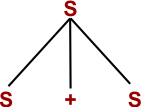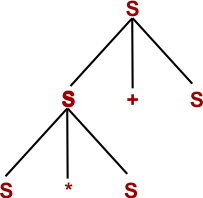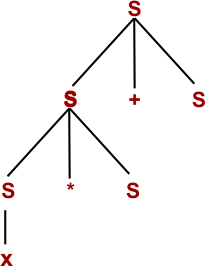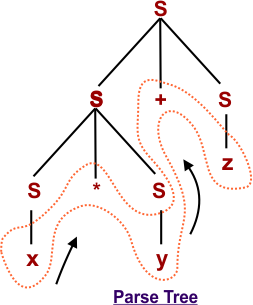Select Page

# Parse Tree in Automata

The process of deriving a string from given grammar is called as derivation. The geometrical representation of a derivation is known as a derivation tree or parse tree.

In Parse Tree, the deepest sub-tree from leftmost is traversed first by following the rule of In-order traversal. In this way the original input string is obtained but

• All leaf nodes must be terminals.
• All interior nodes must be Non-terminals.

## Example of Parse Tree

Suppose the following Production rules of a Grammar (G)

S  S + S | S * S

S x|y|z

and Input is (x * y + Z)

Step 1Step 2Step 3Step 4Step 5Help Other’s By Sharing…